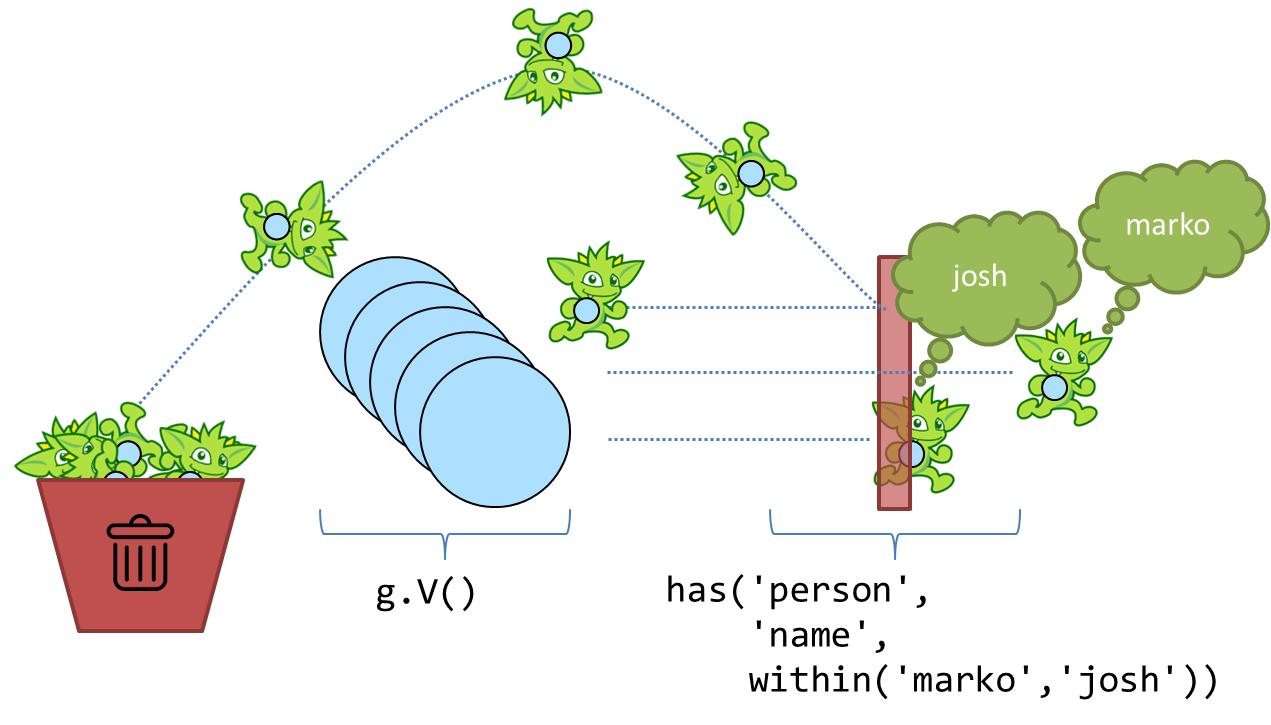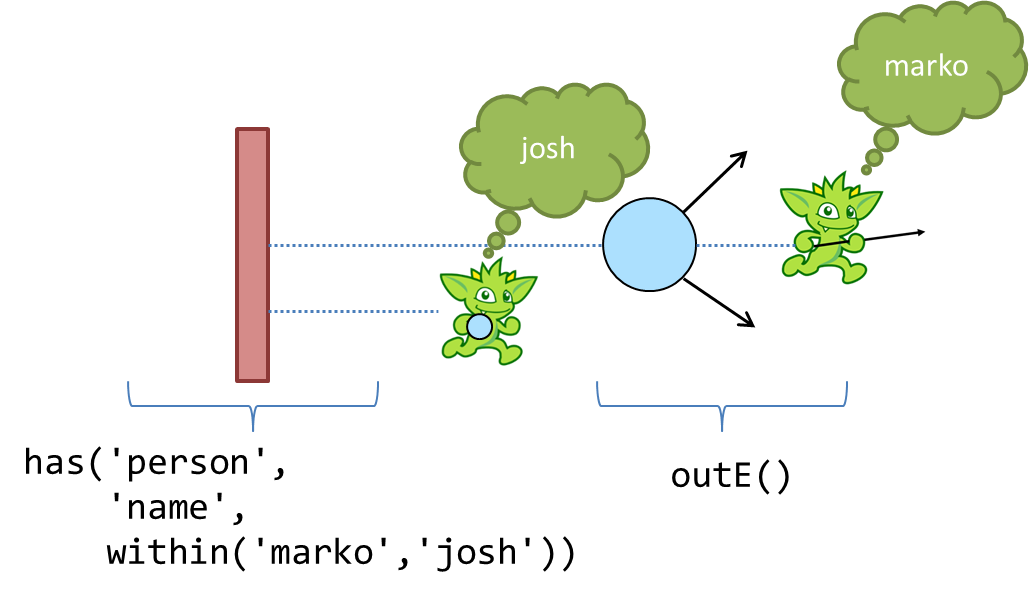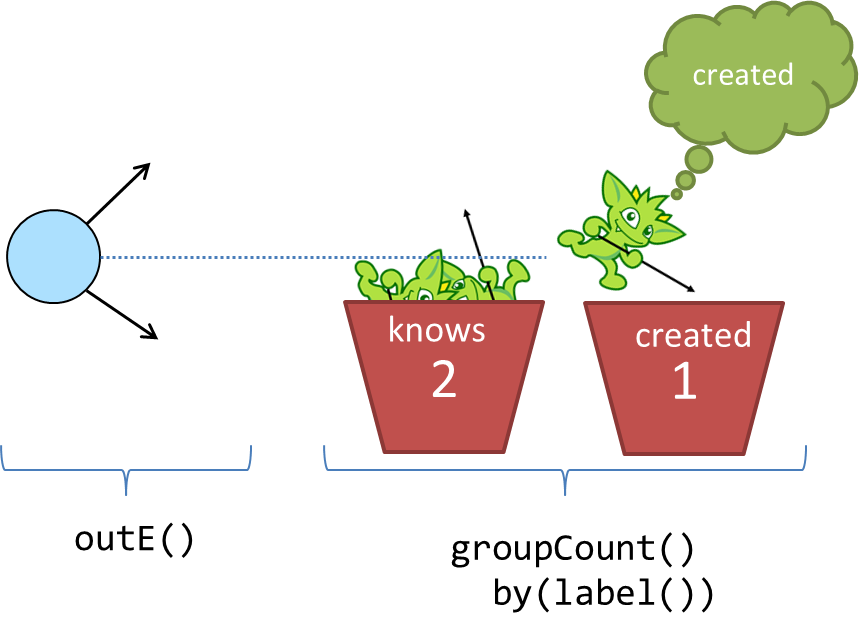3.5.1

## Gremlin’s AnatomyThe Gremlin language is typically described by the individual steps that make up the language, but it is worth taking a look at the component parts of Gremlin that make a traversal work. Understanding these component parts make it possible to discuss and understand more advanced Gremlin topics, such as Gremlin DSL development and Gremlin debugging techniques. Ultimately, Gremlin’s Anatomy provides a foundational understanding for helping to read and follow Gremlin of arbitrary complexity, which will lead you to more easily identify traversal patterns and thus enable you to craft better traversals of your own.

 Note This tutorial is based on Stephen Mallette’s presentation on Gremlin’s Anatomy - the slides for that presentation can be found here.

The component parts of a Gremlin traversal can be all be identified from the following code:

``````gremlin> g.V().
has('person', 'name', within('marko', 'josh')).
outE().
groupCount().
by(label()).next()
==>created=3
==>knows=2``````
``````g.V().
has('person', 'name', within('marko', 'josh')).
outE().
groupCount().
by(label()).next()``````

In plain English, this traversal requests an out-edge label distribution for "marko" and "josh". The following sections, will pick this traversal apart to show each component part and discuss it in some detail.

### GraphTraversalSource

`g.V()` - You are likely well acquainted with this bit of Gremlin. It is in virtually every traversal you read in documentation, blog posts, or examples and is likely the start of most every traversal you will write in your own applications.

``````gremlin> g.V()
==>v
==>v
==>v
==>v
==>v
==>v``````
``g.V()``

While it is well known that `g.V()` returns a list of all the vertices in the graph, the technical underpinnings of this ubiquitous statement may be less so well established. First of all, the `g` is a variable. It could have been `x`, `y` or anything else, but by convention, you will normally see `g`. This `g` is a `GraphTraversalSource` and it spawns `GraphTraversal` instances with start steps. `V()` is one such start step, but there are others like `E` for getting all the edges in the graph. The important part is that these start steps begin the traversal.

In addition to exposing the available start steps, the `GraphTraversalSource` also holds configuration options (perhaps think of them as pre-instructions for Gremlin) to be used for the traversal execution. The methods that allow you to set these configurations are prefixed by the word "with". Here are a few examples to consider:

``````g.withStrategies(SubgraphStrategy.build().vertices(hasLabel('person')).create()).  //1
V().has('name','marko').out().values('name')
g.withSack(1.0f).V().sack()                                                        //2
g.withComputer().V().pageRank()                                                    //3``````
1. Define a strategy for the traversal

2. Define an initial sack value

3. Define a GraphComputer to use in conjunction with a `VertexProgram` for OLAP based traversals - for example, see Spark

 Important How you instantiate the `GraphTraversalSource` is highly dependent on the graph database implementation you are using. Typically, they are instantiated from a `Graph` instance with the `traversal()` method, but some graph databases, ones that are managed or "server-oriented", will simply give you a `g` to work with. Consult the documentation of your graph database to determine how the `GraphTraversalSource` is constructed.

### GraphTraversal

As you now know, a `GraphTraversal` is spawned from the start steps of a `GraphTraversalSource`. The `GraphTraversal` contain the steps that make up the Gremlin language. Each step returns a `GraphTraversal` so that the steps can be chained together in a fluent fashion. Revisiting the example from above:

``````gremlin> g.V().
has('person', 'name', within('marko', 'josh')).
outE().
groupCount().
by(label()).next()
==>created=3
==>knows=2``````
``````g.V().
has('person', 'name', within('marko', 'josh')).
outE().
groupCount().
by(label()).next()``````

the `GraphTraversal` components are represented by the `has()`, `outE()` and `groupCount()`-steps. The key to reading this Gremlin is to realize that the output of one step becomes the input to the next. Therefore, if you consider the start step of `V()` and realize that it returns vertices in the graph, the input to `has()` is going to be a `Vertex`. The `has()`-step is a filtering step and will take the vertices that are passed into it and block any that do not meet the criteria it has specified. In this case, that means that the output of the `has()`-step is vertices that have the label of "person" and the "name" property value of "josh" or "marko".Given that you know the output of `has()`, you then also know the input to `outE()`. Recall that `outE()` is a navigational step in that it enables movement about the graph. In this case, `outE()` tells Gremlin to take the incoming "marko" and "josh" vertices and traverse their outgoing edges as the output.Now that it is clear that the output of `outE()` is an edge, you are aware of the input to `groupCount()` - edges. The `groupCount()`-step requires a bit more discussion of other Gremlin components and will thus be examined in the following sections. At this point, it is simply worth noting that the output of `groupCount()` is a `Map` and if a Gremlin step followed it, the input to that step would therefore be a `Map`.

The previous paragraph ended with an interesting point, in that it implied that there were no "steps" following `groupCount()`. Clearly, `groupCount()` is not the last function to be called in that Gremlin statement so you might wonder what the remaining bits are, specifically: `by(label()).next()`. The following sections will discuss those remaining pieces.

### Step Modulators

It’s been explained in several ways now that the output of one step becomes the input to the next, so surely the `Map` produced by `groupCount()` will feed the `by()`-step. As alluded to at the end of the previous section, that expectation is not correct. Technically, `by()` is not a step. It is a step modulator. A step modulator modifies the behavior of the previous step. In this case, it is telling Gremlin how the key for the `groupCount()` should be determined. Or said another way in the context of the example, it answers this question: What do you want the "marko" and "josh" edges to be grouped by?

### Anonymous Traversals

In this case, the answer to that question is provided by the anonymous traversal `label()` as the argument to the step modulator `by()`. An anonymous traversal is a traversal that is not bound to a `GraphTraversalSource`. It is constructed from the double underscore class (i.e. `__`), which exposes static functions to spawn the anonymous traversals. Typically, the double underscore is not visible in examples and code as by convention, TinkerPop typically recommends that the functions of that class be exposed in a standalone fashion. In Java, that would mean statically importing the methods, thus allowing `__.label()` to be referred to simply as `label()`.

 Note In Java, the full package name for the `__` is `org.apache.tinkerpop.gremlin.process.traversal.dsl.graph`.

In the context of the example traversal, you can imagine Gremlin getting to the `groupCount()`-step with a "marko" or "josh" outgoing edge, checking the `by()` modulator to see "what to group by", and then putting edges into buckets by their `label()` and incrementing a counter on each bucket.The output is thus an edge label distribution for the outgoing edges of the "marko" and "josh" vertices.

### Terminal Step

Terminal steps are different from the `GraphTraversal` steps in that terminal steps do not return a `GraphTraversal` instance, but instead return the result of the `GraphTraversal`. In the case of the example, `next()` is the terminal step and it returns the `Map` constructed in the `groupCount()`-step. Other examples of terminal steps include: `hasNext()`, `toList()`, and `iterate()`. Without terminal steps, you don’t have a result. You only have a `GraphTraversal`.

 Note You can read more about traversal iteration in the Gremlin Console Tutorial.

### Expressions

It is worth backing up a moment to re-examine the `has()`-step. Now that you have come to understand anonymous traversals, it would be reasonable to make the assumption that the `within()` argument to `has()` falls into that category. It does not. The `within()` option is not a step either, but instead, something called an expression. An expression typically refers to anything not mentioned in the previously described Gremlin component categories that can make Gremlin easier to read, write and maintain. Common examples of expressions would be string tokens, enum values, and classes with static methods that might spawn certain required values.

A concrete example would be the class from which `within()` is called - `P`. The `P` class spawns `Predicate` values that can be used as arguments for certain traversal steps. Another example would be the `T` enum which provides a type safe way to reference `id` and `label` keys in a traversal. Like anonymous traversals, these classes are usually statically imported so that instead of having to write `P.within()`, you can simply write `within()`, as shown in the example.

## Conclusion

There’s much more to a traversal than just a bunch of steps. Gremlin’s Anatomy puts names to each of these component parts of a traversal and explains how they connect together. Understanding these component parts should help provide more insight into how Gremlin works and help you grow in your Gremlin abilities.